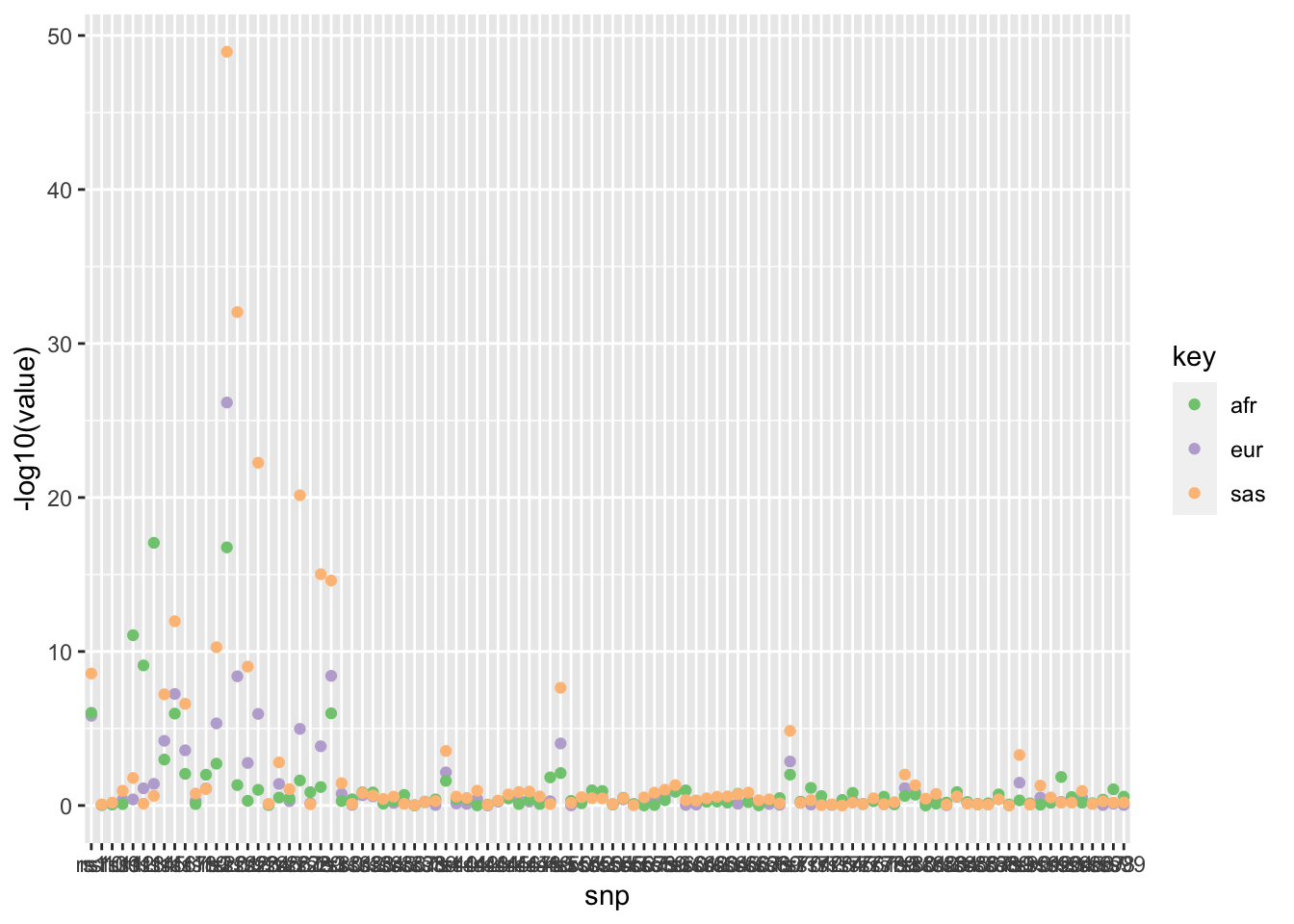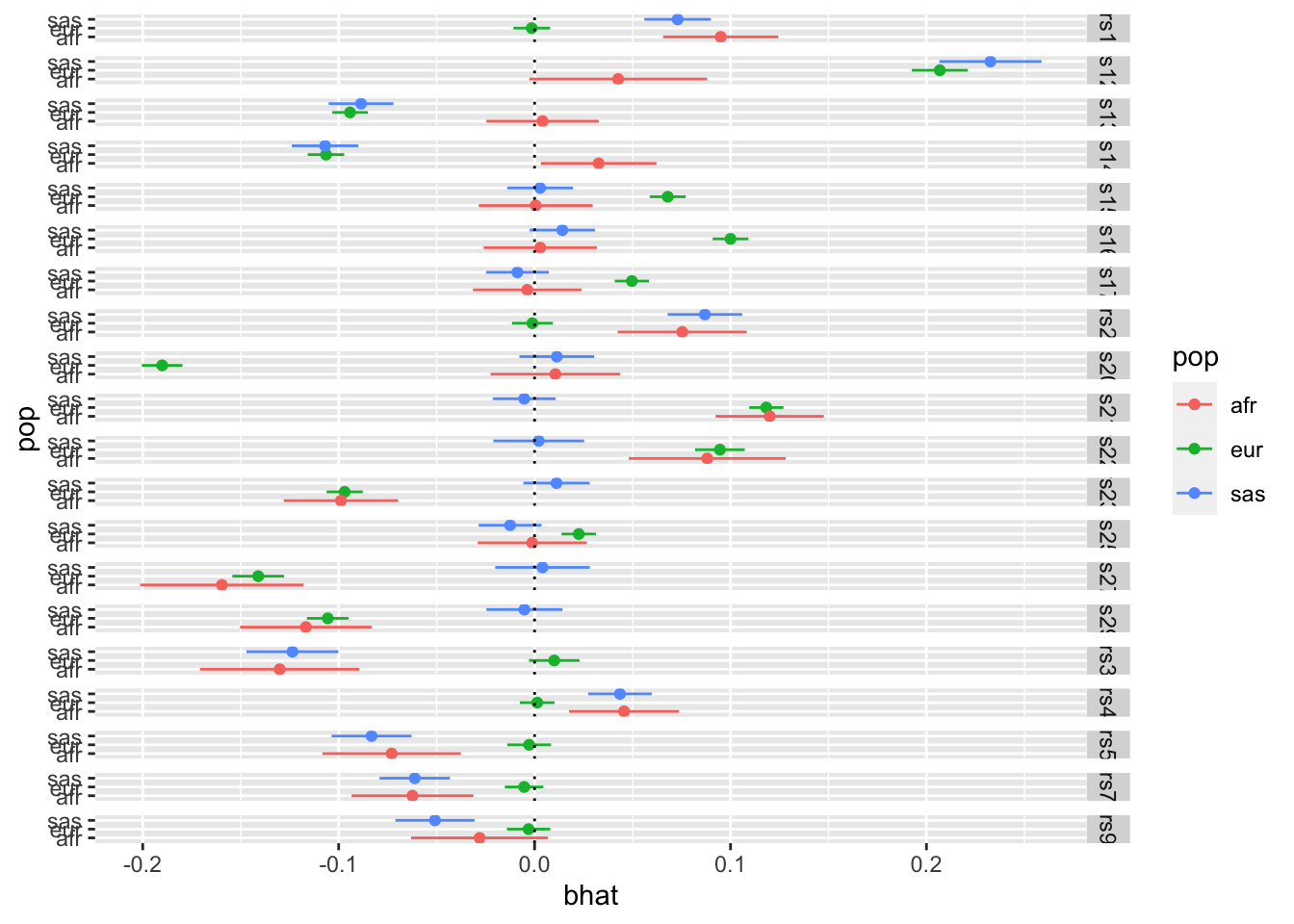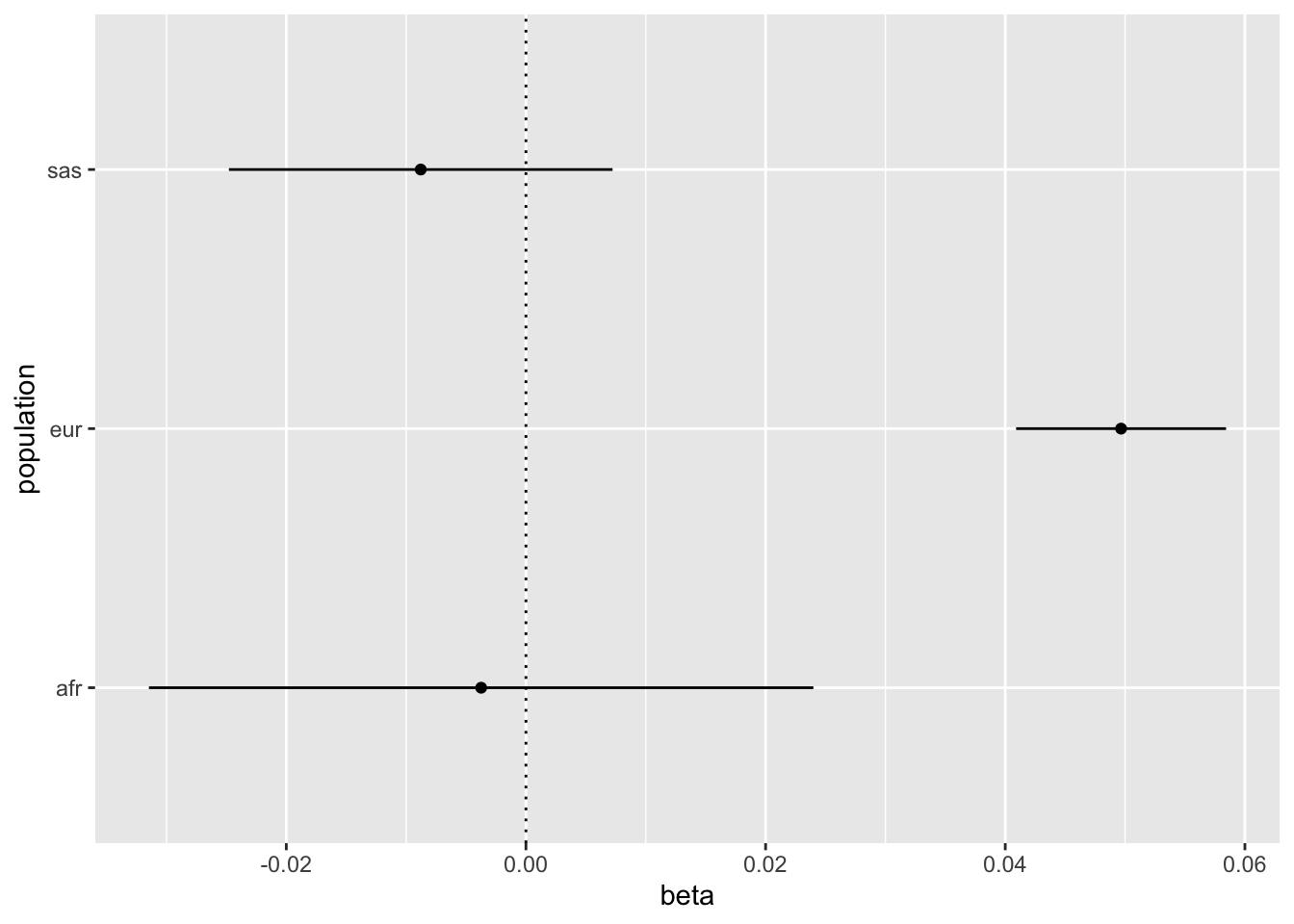# Cross group effect comparison

Author

Gibran Hemani

Published

June 7, 2023

## Background

GWAS being performed on multiple ancestries, then meta-analysing to mitigate problems of LD tagging. QUESTION - how to determine rate of agreement of associations across ancestries. Power differs due to different allele frequencies and sample sizes (reflected in the SE of the assoc).

• Test statistic comparing observed vs replication rates

Simulate 100 effects for 3 populations. For each population select a few SNPs to be null, otherwise all SNPs have the same effect in each population. Each population has a different sample size.

``````library(simulateGP)
library(dplyr)``````
``````
Attaching package: 'dplyr'``````
``````The following objects are masked from 'package:stats':

filter, lag``````
``````The following objects are masked from 'package:base':

intersect, setdiff, setequal, union``````
``````library(tidyr)
library(ggplot2)
map <- tibble(snp=paste0("rs",1:100), af=runif(100, 0.01, 0.99))
params <- generate_gwas_params(map=map, h2=0.2, S=-0.4, Pi=1)

temp <- params; temp\$beta[1:10] <- 0
ss_eur <- generate_gwas_ss(temp, 100000)

temp <- params; temp\$beta[11:20] <- 0
ss_afr <- generate_gwas_ss(temp, 10000)

temp <- params; temp\$beta[15:30] <- 0
ss_sas <- generate_gwas_ss(temp, 30000)``````

So now each population has SNP effect estimates for 100 SNPs, an example of what the dataset looks like:

``ss_eur``
``````# A tibble: 100 × 7
snp      af      se     bhat   fval      n  pval
<chr> <dbl>   <dbl>    <dbl>  <dbl>  <dbl> <dbl>
1 rs1   0.330 0.00476 -0.00147 0.0960 100000 0.757
2 rs2   0.229 0.00532 -0.00110 0.0430 100000 0.836
3 rs3   0.133 0.00658  0.0100  2.33   100000 0.127
4 rs4   0.417 0.00454  0.00134 0.0873 100000 0.768
5 rs5   0.190 0.00570 -0.00276 0.235  100000 0.628
6 rs6   0.362 0.00465  0.00309 0.442  100000 0.506
7 rs7   0.273 0.00502 -0.00535 1.13   100000 0.287
8 rs8   0.497 0.00447  0.00192 0.184  100000 0.668
9 rs9   0.195 0.00564 -0.00308 0.298  100000 0.585
10 rs10  0.426 0.00452  0.00524 1.34   100000 0.246
# ℹ 90 more rows``````

## Heterogeneity

For each SNP test for heterogeneity of effects between populations. This uses Cochrane’s Q test statistic.

Analysis functions:

``````fixed_effects_meta_analysis <- function(beta_vec, se_vec)
{
w <- 1 / se_vec^2
beta <- sum(beta_vec * w) / sum(w)
se <- sqrt(1 / sum(w))
pval <- pnorm(abs(beta / se), lower.tail = FALSE)
Qj <- w * (beta-beta_vec)^2
Q <- sum(Qj)
Qdf <- length(beta_vec)-1
Qjpval <- pchisq(Qj, 1, lower.tail=FALSE)
Qpval <- pchisq(Q, Qdf, lower.tail=FALSE)
return(list(beta=beta, se=se, Qpval=Qpval, Qj=Qj, Qjpval=Qjpval))
}
# fixed_effects_meta_analysis(c(1,2,3), c(0.3, 0.3, 0.3))
# fixed_effects_meta_analysis(c(1,1,1), c(0.3, 0.3, 0.3))

#' Test for heterogeneity of effect estimates between populations
#'
#' @description For each SNP this function will provide a Cochran's Q test statistic - a measure of heterogeneity of effect sizes between populations. A low p-value means high heterogeneity.
#' In addition, for every SNP it gives a per population p-value - this can be interpreted as asking for each SNP is a particular giving an outlier estimate.
#'
#' @param sslist Named list of data frames, one for each population, with at least bhat, se and snp columns
#'
#' @return List
#' - Q = vector of p-values for Cochrane's Q statistic for each SNP
#' - Qj = Data frame of per-population outlier q values for each SNP
heterogeneity_test <- function(sslist)
{
b <- lapply(sslist, \(x) x\$bhat) %>% bind_cols
se <- lapply(sslist, \(x) x\$se) %>% bind_cols
o <- lapply(1:nrow(b), \(i) {
fixed_effects_meta_analysis(as.numeric(b[i,]), as.numeric(se[i,]))
})
Q <- tibble(snp = sslist[]\$snp, Qpval = sapply(o, \(x) x\$Qpval))
Qj <- lapply(o, \(x) x\$Qjpval) %>% do.call(rbind, .) %>%
as_tibble() %>%
rename(setNames(paste0("V", 1:length(sslist)), names(sslist))) %>%
mutate(snp = sslist[]\$snp)
return(list(Q=Q, Qj=Qj))
}``````

Run analysis:

``o <- heterogeneity_test(list(eur=ss_eur, afr=ss_afr, sas=ss_sas))``
``````Warning: The `x` argument of `as_tibble.matrix()` must have unique column names if
`.name_repair` is omitted as of tibble 2.0.0.
ℹ Using compatibility `.name_repair`.``````
``o\$Q``
``````# A tibble: 100 × 2
snp      Qpval
<chr>    <dbl>
1 rs1   1.19e-18
2 rs2   1.00e-16
3 rs3   4.70e-27
4 rs4   3.01e- 6
5 rs5   2.31e-12
6 rs6   1.31e- 2
7 rs7   1.75e- 8
8 rs8   3.59e- 3
9 rs9   1.94e- 4
10 rs10  9.73e- 1
# ℹ 90 more rows``````
``````ggplot(o\$Q, aes(x=snp, y=-log10(Qpval))) +
geom_point() +
geom_hline(yintercept=-log10(0.05/nrow(o\$Q)))``````This shows the heterogeneity for each SNP. Alternatively we could look at the contribution of each population to the heterogeneity of each SNP

``````gather(as.data.frame(o\$Qj), "key", "value", -snp) %>%
ggplot(., aes(x=snp, y=-log10(value))) +
geom_point(aes(colour=key)) +
scale_colour_brewer(type="qual")``````We could then dig deeper and look at the per-population estimates for the SNPs that have some heterogeneity

``````# Which SNPs have heterogeneity after multiple testing correction
index <- which(o\$Q\$Qpval < 0.05/nrow(o\$Q))

# Get per-population effect estimates for those SNPs
# Make forest plots
bind_rows(
ss_eur[index,] %>% mutate(pop="eur"),
ss_afr[index,] %>% mutate(pop="afr"),
ss_sas[index,] %>% mutate(pop="sas")
) %>% ggplot(., aes(x=bhat, y=pop)) +
geom_point(aes(colour=pop)) +
geom_errorbarh(aes(colour=pop, xmin=bhat-se*1.96, xmax=bhat+se*1.96), height=0) +
facet_grid(snp ~ .) +
geom_vline(xintercept=0, linetype="dotted")``````## Expected vs observed replication

This might not be as useful as the heterogeneity stuff above, but some example code below

``````#' Expected vs observed replication rates
#'
#' @description For a set of effects that have discovery and replication betas and SEs, this function determines the extent to which the observed replication rate matches the expected replication rate.
#' The expected replication rate is based on the assumption that the replication dataset has the same effect sizes but that the power may be different (e.g. due to allele frequencies or sample sizes) and is reflected in the replication standard errors.
#' It assesses replication based on concordance of effect direction across discovery and replication, and p-values surpassing a user-specified p-value threshold.
#'
#' @param b_disc vector of clumped incidence hit effects
#' @param se_disc the standard errors for incidence effects
#' @param b_rep corresponding vector of associations in progression
#' @param se_rep standard errors of effects in progression
#' @param alpha p-value threshold to check for replication of incidence hits in progression (e.g. try 0.05 or 1e-5)
expected_vs_observed_replication <- function(b_disc, b_rep, se_disc, se_rep, alpha)
{
p_sign <- pnorm(-abs(b_disc) / se_disc) * pnorm(-abs(b_disc) / se_rep) + ((1 - pnorm(-abs(b_disc) / se_disc)) * (1 - pnorm(-abs(b_disc) / se_rep)))
p_sig <- pnorm(-abs(b_disc) / se_rep + qnorm(alpha / 2)) + (1 - pnorm(-abs(b_disc) / se_rep - qnorm(alpha / 2)))
p_rep <- pnorm(abs(b_rep)/se_rep, lower.tail=FALSE)
res <- tibble::tibble(
nsnp=length(b_disc),
metric=c("Sign", "Sign", "P-value", "P-value"),
datum=c("Expected", "Observed", "Expected", "Observed"),
value=c(sum(p_sign, na.rm=TRUE), sum(sign(b_disc) == sign(b_rep)), sum(p_sig, na.rm=TRUE), sum(p_rep < alpha, na.rm=TRUE)),
pdiff=c(NA_real_, binom.test(value, nsnp, value/nsnp)\$p.value, NA_real_, binom.test(value, nsnp, value/nsnp)\$p.value)
)
res_per_variant <- tibble(
expected_pval = p_sig,
observed_pval = p_rep < alpha,
replication_fail = expected_pval > 0.95 & ! observed_pval,
expected_sign = p_sign,
observed_sign = sign(b_disc) == sign(b_rep),
sign_fail = expected_sign > 0.95 & ! observed_sign
)
return(list(res=res, variants=res_per_variant))
}

forest_plot <- function(sslist, snp)
{
tibble(
beta = sapply(sslist, \(x) x\$bhat[snp]),
se = sapply(sslist, \(x) x\$se[snp]),
label = names(sslist)
) %>%
ggplot(., aes(x=beta, y=label)) +
geom_point() +
geom_errorbarh(aes(xmin=beta-se*1.96, xmax=beta+se*1.96), height=0) +
geom_vline(xintercept=0, linetype="dotted") +
labs(x="beta", y="population")
}``````
``````index <- which(ss_eur\$pval < 5e-8)
o_eur_afr <- expected_vs_observed_replication(ss_eur\$bhat[index], ss_afr\$bhat[index], ss_eur\$se[index], ss_afr\$se[index], 0.05)
o_eur_afr``````
``````\$res
# A tibble: 4 × 5
nsnp metric  datum    value       pdiff
<int> <chr>   <chr>    <dbl>       <dbl>
1    59 Sign    Expected  58.7 NA
2    59 Sign    Observed  54    0.00000976
3    59 P-value Expected  52.1 NA
4    59 P-value Observed  48    0.103

\$variants
# A tibble: 59 × 6
expected_pval observed_pval replication_fail expected_sign observed_sign
<dbl> <lgl>         <lgl>                    <dbl> <lgl>
1         1.00  TRUE          FALSE                    1     TRUE
2         1.00  FALSE         TRUE                     1.00  FALSE
3         1.00  TRUE          FALSE                    1.00  FALSE
4         0.996 FALSE         TRUE                     1.00  TRUE
5         1.00  FALSE         TRUE                     1.00  TRUE
6         0.940 FALSE         FALSE                    1.00  FALSE
7         0.765 FALSE         FALSE                    0.996 FALSE
8         1     FALSE         TRUE                     1     FALSE
9         1.00  TRUE          FALSE                    1     TRUE
10         0.996 TRUE          FALSE                    1.00  TRUE
# ℹ 49 more rows
# ℹ 1 more variable: sign_fail <lgl>``````
``forest_plot(list(eur=ss_eur, afr=ss_afr, sas=ss_sas), index[which(o_eur_afr\$variants\$sign_fail)])````````index <- which(ss_afr\$pval < 5e-8)
o_afr_sas <- expected_vs_observed_replication(ss_afr\$bhat[index], ss_afr\$bhat[index], ss_sas\$se[index], ss_sas\$se[index], 0.05)
o_afr_sas``````
``````\$res
# A tibble: 4 × 5
nsnp metric  datum    value pdiff
<int> <chr>   <chr>    <dbl> <dbl>
1    21 Sign    Expected    21    NA
2    21 Sign    Observed    21     1
3    21 P-value Expected    21    NA
4    21 P-value Observed    21     1

\$variants
# A tibble: 21 × 6
expected_pval observed_pval replication_fail expected_sign observed_sign
<dbl> <lgl>         <lgl>                    <dbl> <lgl>
1             1 TRUE          FALSE                        1 TRUE
2             1 TRUE          FALSE                        1 TRUE
3             1 TRUE          FALSE                        1 TRUE
4             1 TRUE          FALSE                        1 TRUE
5             1 TRUE          FALSE                        1 TRUE
6             1 TRUE          FALSE                        1 TRUE
7             1 TRUE          FALSE                        1 TRUE
8             1 TRUE          FALSE                        1 TRUE
9             1 TRUE          FALSE                        1 TRUE
10             1 TRUE          FALSE                        1 TRUE
# ℹ 11 more rows
# ℹ 1 more variable: sign_fail <lgl>``````

``sessionInfo()``
``````R version 4.3.0 (2023-04-21)
Platform: aarch64-apple-darwin20 (64-bit)
Running under: macOS Monterey 12.6.2

Matrix products: default
BLAS:   /Library/Frameworks/R.framework/Versions/4.3-arm64/Resources/lib/libRblas.0.dylib
LAPACK: /Library/Frameworks/R.framework/Versions/4.3-arm64/Resources/lib/libRlapack.dylib;  LAPACK version 3.11.0

locale:
 en_US.UTF-8/en_US.UTF-8/en_US.UTF-8/C/en_US.UTF-8/en_US.UTF-8

time zone: Europe/London
tzcode source: internal

attached base packages:
 stats     graphics  grDevices utils     datasets  methods   base

other attached packages:
 ggplot2_3.4.2    tidyr_1.3.0      dplyr_1.1.2      simulateGP_0.1.2

loaded via a namespace (and not attached):
 vctrs_0.6.2        cli_3.6.1          knitr_1.43         rlang_1.1.1
 xfun_0.39          purrr_1.0.1        generics_0.1.3     jsonlite_1.8.4
 labeling_0.4.2     glue_1.6.2         colorspace_2.1-0   htmltools_0.5.5
 scales_1.2.1       fansi_1.0.4        rmarkdown_2.22     grid_4.3.0
 munsell_0.5.0      evaluate_0.21      tibble_3.2.1       fastmap_1.1.1
 yaml_2.3.7         lifecycle_1.0.3    compiler_4.3.0     RColorBrewer_1.1-3
 htmlwidgets_1.6.2  pkgconfig_2.0.3    rstudioapi_0.14    farver_2.1.1
 digest_0.6.31      R6_2.5.1           tidyselect_1.2.0   utf8_1.2.3
 pillar_1.9.0       magrittr_2.0.3     withr_2.5.0        gtable_0.3.3
 tools_4.3.0       ``````